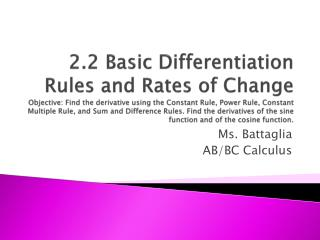DownloadDownload PresentationMs. Battaglia AB/BC Calculus

# Ms. Battaglia AB/BC Calculus

Télécharger la présentation## Ms. Battaglia AB/BC Calculus

- - - - - - - - - - - - - - - - - - - - - - - - - - - E N D - - - - - - - - - - - - - - - - - - - - - - - - - - -
##### Presentation Transcript

1. 2.2 Basic Differentiation Rules and Rates of ChangeObjective: Find the derivative using the Constant Rule, Power Rule, Constant Multiple Rule, and Sum and Difference Rules. Find the derivatives of the sine function and of the cosine function. Ms. Battaglia AB/BC Calculus

2. Thm 2.2 The Constant Rule The derivative of a constant function is 0. That is, if c is a real number, then Example: FunctionDerivative y = 10 dy/dx = f(x) = 0 f’(x) = s(t) = -2 s’(t) = y = k, k is constant y’ =

3. Thm 2.3 The Power Rule If n is a rational number, then the function is differentiable and For f to be differentiable at x=0, n must be a number such that is defined on an interval containing 0.

4. Using the Power Rule a. b. c.

5. Finding the Slope of a Graph Find the slope of the graph of when a. x = 0 b. x = 1 c. x = 4

6. Finding an Equation of a Tangent Line • Find an equation of the tangent line to the graph of when x=1.

7. Thm 2.4 The Constant Multiple Rule If f is a differentiable function and c is a real number, then cf is also differentiable and . Thm 2.5 The Sum and Difference Rules The sum (or difference) of two differentiable functions f and g is itself differentiable. Moreover, the derivative of f+g (or f-g) is the sum (or difference) of the derivatives of f and g.

8. Using the Constant Multiple Rule a. b. c.

9. Using the Constant Multiple Rule d. e. y

10. Using Parenthesis When Differentiating

11. Using the Sum and Difference Rules a. b.

12. Derivatives of the Sine & Cosine Functions Theorem 2.6

13. Derivatives Involving Sines & Cosines a. + 7 b. c.

14. Example • Determine the point(s) (if any) at which the graph of has a horizontal tangent line.

15. Rates of Change the average velocity is

16. Classwork/Homework • AB: Page 116 #59-65 odd, 79, 107, 110, 111, 113, 117,119, graphing worksheet • BC: Page 116 #59-65 odd, 79, 107, 110, 111, 113, 117,119, AP sample problem worksheet